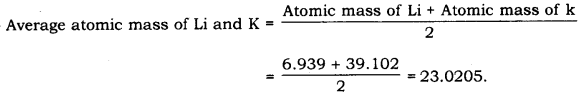# On the basis of Mendeleev’s Periodic Table given below, answer the questions that follow the table:

On the basis of Mendeleev’s Periodic Table given below, answer the questions that follow the table:

1. Name the element which is in
(i). Ist group and 3rd period.
(ii). VIIth group and 2nd period.

2. Suggest the formula for the following:
(i). oxide of nitrogen.
(ii). hydride of oxygen.

3. In group VIII of the Periodic Table, why does cobalt with atomic mass 58.93 appear before nickel having atomic mass 58.71?

4. Besides gallium, which two other elements have since been discovered for which Mendeleev had left gaps in his periodic table?

5. Using atomic masses of Li, Na and K, find the average atomic mass of Li and K and compare it with the atomic of Na. State the conclusion drawn from this activity.

1
(i). The elements present in the Ist group of the periodic table are H, Li, Na, K, Rb and Cs. The only element that belong to the first group and third period is sodium (Na).

(ii). The elements present in the VIIth group are F, Cl, Br and I. The only element that belongs to the VIIth group and 2nd period is fluorine (F).

2
(i). Nitrogen belongs the Vth group of the periodic table. The elements belonging to the
Vth group from oxides having the general formula R2Os. Thus, the formula of the oxide formed by nitrogen is N2C>5.

(ii). Oxygen belong to the VIth group of the periodic table. The elements belonging to the VIth group form hydrides having the general formula RHr Thus, the formula of the hydride formed by oxygen is OH2 or H2O.

3.Elements with similar chemical properties are placed in the same group of the periodic table. Cobalt (CO)2 rhodium (Rh) and iridium (lr) have similar chemical properties. In group VIIIth of the periodic table. Cobalt with atomic mass 58.93 appears before nickel having atomic mass 58.71 so that, the elements with similar chemical properties may fall in the same group.

4.Scandium (Sc) and germanium (Ge)

5.From Mendeleev’s Periodic Table, the atomic masses of Li, Na and K are found to be 6.939, 22.99 and 39.102 respectively.